• 在矩阵中寻找最大正方形连续区域问题描叙 输入一个矩阵M、一个数字k，找出一个最大的正方形连续区域，这个区域里的数字均是k。 ​ 界的思考 对于矩阵M的每一个元素，要么等于k要么不等于k，要知道这个数的...
在矩阵中寻找最大正方形连续区域

问题描叙

输入一个矩阵M、一个数字k，找出一个最大的正方形连续区域，这个区域里的数字均是k。  ​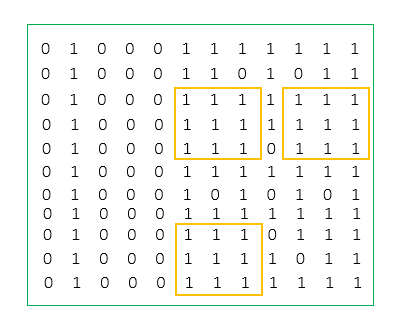界的思考

对于矩阵M中的每一个元素，要么等于k要么不等于k，要知道这个数的状态，必须有一次比较。一共n个数，所以至少需要

n2
$n^2$次比较。故比较次数的下界为

Ω(n2)
$\Omega(n^2)$，那么是否存在一个

O(n2)
$O(n^2)$的算法来解决这个问题呢？

算法

M中的每一个元素

Mij
$M_{ij}$都有一个与之对应的数值

maxij
$max_{ij}$记录着以这个元素为左上角顶点的最大的一个正方形连续区域的大小(行/列 数)。  对元素

Mij
$M_{ij}$有两种状态

Mij≠k
$M_{ij}\ne k$ , 此时可以得出

maxij=0
$max_{ij}=0$。

Mij=k
$M_{ij} = k$ , 此时可以得出

maxij=min(max(i+1)j,maxi(j+1),max(i+1)(j+1))+1
$max_{ij}=min(max_{(i+1)j},max_{i(j+1)},max_{(i+1)(j+1)})+1$。
​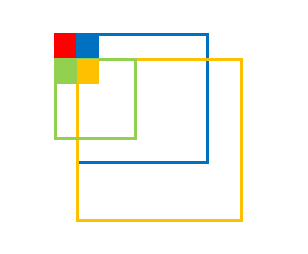从右到左，从下往上地扫描矩阵M，计算出每一个元素的

maxij
$max_{ij}$，其中最大的

maxij
$max_{ij}$就是矩阵M的最大正方形连续区域的大小，其对应的元素的下标(i,j)就是这块连续区域的左上角顶点。

内存使用

大可不必创建

row×column
$row \times column$ 个空间来存储每一个元素的

maxij
$max_{ij}$，实际上，在比较过程中只用

min(row,column)+1
$min(row,column)+1$ 个内存空间就行了。

过程图示

例图:  ​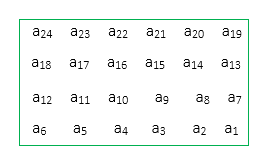第一轮循环:
​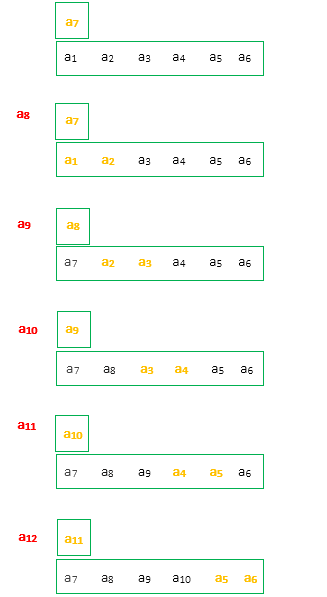第二轮循环:
​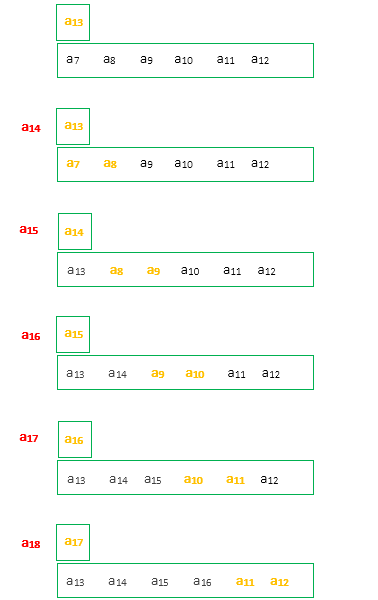第三轮循环:
​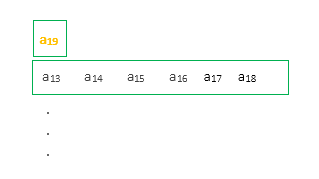用一个数据结构记录当前找到的最大的连续正方形的大小和对应的左上角坐标。
在比较的过程中，如果遇到更大的连续正方形，则更新记录。

代码
#include<iostream>

using namespace std;

struct max_memo{//记录最大连续正方形区域的大小和左上角的坐标

//左上角的坐标
int row;
int col;

int num;//大小---以行or列为单位

};

//放回三个数中最小值。
int min(int left, int down, int leftdown){

left = left < down ? left : down;

return  left < leftdown ? left : leftdown;
}
< search >
void search(int M[],int k,int row,int col, max_memo &Max_memo){

int *memo = new int[row];
int up_one;
int current;

for (int i(0); i <row; i++){//初始化最后一行
if (M[col - 1][row - 1 - i] == k)
memo[i] = 1;
else memo[i] = 0;
}

Max_memo.col = col - 1, Max_memo.row = row - 1, Max_memo.num = memo;//初始化

int ex_index;//记录交换时的下标。

for (int row_local(row - 2), col_local(col - 1); row_local >= 0;)//外循环。
{

col_local = (col - 1);//初始化列坐标---每次内循环从最右边开始，向左移动。

if (M[row_local][col_local] == k) {
up_one = 1;//计算最右端的元素的最大连续区域（0 or 1）

if (up_one > Max_memo.num){
Max_memo.col = col_local, Max_memo.row = row_local, Max_memo.num = up_one;//更新
}
}
else up_one = 0;

col_local--;

while (col_local >= 0){//内循环

ex_index = (col - 1) - col_local-1;

if (M[row_local][col_local] != k){
current = 0;
}

if (M[row_local][col_local] == k){

current = min(up_one, memo[ex_index + 1], memo[ex_index]) + 1;
if (current > Max_memo.num){
Max_memo.col = col_local, Max_memo.row = row_local, Max_memo.num = current;//更新
}
}

memo[ex_index] = up_one;
up_one = current;

col_local--;//向左移
}

memo[row - 1] = up_one;//第一轮循环结束。

row_local--;//向上移
}

delete[] memo;
}
< main >
int main(){

int M[] = {
/*  { 0, 1, 1, 1, 1 },
{ 0, 1, 2, 2, 1 },
{ 1, 1, 2, 2, 1 },
{ 1, 1, 2, 2, 1 },
{ 1, 1, 0, 0, 1 }
*/
{0,0,0,0,1},
{0,0,0,0,1},
{0,0,0,0,1},
{0,0,0,0,1},
{0,0,0,0,0}
};

max_memo Max_memo;

int k = 1;

search(M, k, 5, 5, Max_memo);

if (Max_memo.num)
cout << "结果: 坐标(" << Max_memo.row << ", " << Max_memo.col << "); 大小(以行为单位): " << Max_memo.num << endl;
else cout << "没有值为"<<k<<"的连续正方形区域" << endl;

cout << endl;
system("pause");

}
展开全文• 题目：原题链接（困难） 标签：排序、数学 解法 时间复杂度 空间复杂度 执行用时 Ans 1 (Python) O(L2logL2)O(L^2logL^2)O(L2logL2) ... def maximumNumberOfOnes(self, width: int, height: int, length
题目：原题链接（困难）
标签：排序、数学
解法时间复杂度空间复杂度执行用时Ans 1 (Python)

O

(

L

2

l

o

g

L

2

)

O(L^2logL^2)

O

(

L

2

)

O(L^2)

60ms (57.14%)Ans 2 (Python)Ans 3 (Python)
解法一：
class Solution:
def maximumNumberOfOnes(self, width: int, height: int, length: int, max_ones: int) -> int:
nums = []
for i in range(length):
for j in range(length):
a1, b1 = divmod(width, length)
a2, b2 = divmod(height, length)
c1 = a1 + (1 if b1 > i else 0)
c2 = a2 + (1 if b2 > j else 0)
nums.append(c1 * c2)
nums.sort(reverse=True)
return sum(nums[:max_ones])

展开全文leetcode python 排序 数学
• Matlab 的内置稀疏矩阵乘法函数会不应该发生的情况下导致内存不足异常。 这个文件可以节省一天！ 用“mex mex_amub.cpp”编译它。matlab
• 数值1 在矩阵中最大数量是多少，现有一个尺寸为sideLength*sideLength 的矩阵M,矩阵中的每个单元格的值不是0就是1，而且知道矩阵 的边长maxOnes，计算矩阵中最多可以有多少个1 参考：//...
数值1 在矩阵中的最大数量是多少，现有一个尺寸为sideLength*sideLength 的矩阵M,矩阵中的每个单元格的值不是0就是1，而且知道矩阵 的边长maxOnes，计算矩阵中最多可以有多少个1 参考：//https://leetcode.com/savevmk 根本看不懂
class Solution(object):
def maximumNumberOfOnes(self,width: int,height: int, sideLength:int,maxOnes:int) -> int:
arr = [0 for _ in range(sideLength*sideLength)]
for i in range(height):
for j in range(width):
row = i%sideLength
col = j%sideLength
arr[row*sideLength +col]+=1
arr.sort()# 升序
ans=0
for i in range(maxOnes):
ans += arr[len(arr) -1 -i] # 1的个数
return ans

if __name__ == '__main__':
width,height,sideLength,maxOnes = 2,1,4,2
print(Solution().maximumNumberOfOnes(width,height,sideLength,maxOnes))

展开全文• 35. 求一个矩阵中最大的二维矩阵(元素和最大).如: 1 2 0 3 4 2 3 4 5 1 1 1 5 3 0 中最大的是: 4 5 5 3 要求:(1)写出算法;(2)分析时间复杂度;
• 主要介绍了Python使用min、max函数查找二维数据矩阵中最小、最大值的方法,涉及Python矩阵生成、遍历、查找等相关操作技巧,需要的朋友可以参考下
• ## 求子矩阵的最大和

千次阅读 2016-08-19 22:25:42
来自牛客网左程云第三课第一题 ...矩阵矩阵中的每一个点作为子矩阵的左上角，我们可以得到N*N的数量级的子矩阵数量。那么一共有N*N个点可以作为子矩阵的左上角，子矩阵的数量到达O(N^4)的数量级，我们计算每
来自牛客网左程云第三课第一题
问题：给定一个无序矩阵，其中有正，有负，有 0，求子矩阵的最大和。
要求：时间复杂度O(N^3)
分析：一个最直接的方法就是找到所有的子矩阵，然后遍历这些子矩阵并计算其累加和，找出最大的。矩阵将矩阵中的每一个点作为子矩阵的左上角，我们可以得到N*N的数量级的子矩阵数量。那么一共有N*N个点可以作为子矩阵的左上角，子矩阵的数量到达O(N^4)的数量级，我们在计算每个子矩阵的时候累加和的时候需要遍历每一个数，达到O(N*N)的数量级，整体的时间复杂度达到O(N^6)。子矩阵我们都可以转换成子数组解决，这道题的算法原型是leetcode 53. Maximum Subarray 子数组最大和。
解法：我们求出以每一行的为首行的子矩阵的列累加和，就是将列对应相加，这样我们就得到了一个数组，接下来我们求这个数组的子数组最大和也就是求出了这个子矩阵的最大和。那么我们一共有N行，以这些行为首行的子矩阵有N*N个，求子数组最大和的时间复杂度为O(N)，整体的时间复杂度为O(N^3)。算法原型真的是很重要啊。

public class Main {
public static int maxSum(int[][] m) {
if (m == null || m.length == 0 || m.length == 0) {
return 0;
}
int max = Integer.MIN_VALUE;
for (int i = 0; i < m.length; i++) {
for (int j = i; j < m.length; j++) {
int sum = 0;
for (int k = 0; k < m[j].length; k++) {
if (i != j) {
m[i][k] += m[j][k];// 把累加结果保存在当前行
}
sum += m[i][k];
max = Math.max(max, sum);
sum = sum > 0 ? sum : 0;
}
}
}
return max;
}

public static void main(String[] args) {
int[][] matrix = { { -90, 48, 78 }, { 64, -40, 64 }, { -81, -7, 66 } };
System.out.println(maxSum(matrix));

}
}


展开全文算法
•  给定一个指定的矩阵，维数小于1000，在矩阵的所有子数组寻找具有最大和的子数组求和输出 思路：  典型的动态规划问题 下面是具体的实现： import java.util.Scanner; class largestSubSum { public static ...
• 题目的描述很简单，一个M * N的矩阵中，所有的元素只有0和1， 找出只包含1的最大矩形。 例如：图是一个４　×　６的矩形，画出红色的是我们要找到的区域。 最开始见过这个题目是看一个人写的面经...matrix struct c++ 算法
• 直方图每一项的高度就是从底面行往上走1的数量。我们根据 直方图最大面积的思路 可以求出当前行作为矩阵下边缘的一个最大矩阵。遍历每一行，都能求得其作为底面的矩阵最大面积。 假如矩阵是下图这样的，结果是...
• 题目：给定一个整型矩阵map,其中的值只有0和1两种，求其中全是1的所有矩形区域最大的矩形区域为1的数量。 输出： 6   思路：以每一行做切割，统计以当前行作为底的情况下，每个位置往上的连续1的数量，...
•  矩形的高度可以用数组表示，同时这个场景可以和另外一个场景联系起来：给定一个二维矩阵矩阵中的元素只有0和1，求一个最大的子矩阵(要求子矩阵的元素全是1) ,最大指的是矩阵中的1的数量最多。下图矩阵 a ...
• 矩阵中战斗力最弱的 K 行 一、题目 给你一个大小为 m * n 的矩阵 mat，矩阵由若干军人和...军人 总是 排一行的靠前位置，也就是说 1 总是出现 0 之前。 示例： 输入：mat = [[1,1,0,0,0], [1,1,1,1,0], [1,0,0,0LeetCode 数据结构 算法
• 最大10的数的索引（位置），可先按降序排序，得到索引号，然后将前10个取出即可。 建议方法：order(x,decreasing=TRUE)[1:10] 过程详解： 1、测试数据x> x  0.00 0.00 0.00 0.00 0.00 0.00 0.06 0.09 0.20 ...r语言
• 三个零是最大零，以便夸克质量矩阵中具有违反CP的相位。 然后，保留了六个实际参数和一个违反CP的阶段，这是再现下夸克质量和CKM参数的观测数据所需的最小数目。 检查带有三个零的20个纹理。 其中，有13种纹理...
• ## np中的矩阵

万次阅读 2020-05-01 10:13:07
NumPy，每一个线性的数组称为是一个轴（axes），秩其实是描述轴的数量。比如说，二维数组相当于是一个一维数组，而这个一维数组每个元素又是一个一维数组。所以这个一维数组就是NumPy的轴（axes），而轴的...
• ## 矩阵

千次阅读 2012-09-09 11:15:21
矩阵 维基百科，自由的百科全书 线性代数 向量 · 矩阵 · 行列式 · 线性空间 显示▼向量 显示▼矩阵与行列式 显示...算法 图形 图像处理 汇编
• 输入一个二维01矩阵，判断矩阵中全为1的正方形的最大边长 输入如下：第一行输入...典型的DP问题，把矩阵阵的每个点作为正方形右下角点来处理，则以该点为右下角点的正方形的最大边长，最多比以它的左方、上方和...华为机试题 动态规划 算法
• 思想：将矩阵看做一个二维数组，用scanf()函数输入矩阵，将数组首位设置为最大值max，将max与数组数按顺序两两比较，更新max,比较到最后一位得到最终max。void main(){ int a,i,j,max,max_i,max_j; printf...
• 寻找0 ，1矩阵中含有连续全1块的个数（岛的个数）和0， 1矩阵中最大连续的全1块。 定义 0 ，1矩阵中含有连续全1块的个数 一个矩阵中只有0和1两种值， 每个位置都可以和自己的上、 下、 左、 右 四个位置相连， 如果...
• LARGESTMAX(X)，其中 X 是任意数量的向量和/或矩阵，是这些结构任何一个存在的最大组件。 LARGESTMAX(X) 与 MATLAB 的内置 MAX(X) 一致，仅用于向量！！ 矩阵的 LARGESTMAX(X) 返回等效于 MAX(MAX(X))。 ...matlab
• 我们寻找所有导致夸克质量矩阵中纹理为零且标准模型框架内包含最少数量参数的弱碱。 由于存在十个物理观测值，即六个不消失的夸克质量，三个混合角和一个CP相，因此两个夸克扇区纹理零的最大数目总共为九个。 九...
• 给定一个无序矩阵，其中只有1和0两种值，求只含有1的最大正方形的大小。 例如给定如下矩阵: 1 0 1 0 0 1 0 1 1 1 1 1 1 1 1 1 0 0 1 0 Return 4 int max_square(vector>& matrix) { int row = matrix....
• 给定一个矩阵，该矩阵中的元素只包含1和0，找出该矩阵中全为1的矩形面积的最大值。 如： 1 1 0 0 0 1 0 0 0 1 1 0 0 0 1 对应的矩形面积的最大值为3。 整体思路： 通过求解每列到当前行的连续1的个数，然后...
• 给定一个无序矩阵，其中只有1和0两种值， 求只含有1 的最大的子矩阵大小， 矩阵的大小用其中的元素个数来表示 解题思路： 矩阵压缩， 计算出以每一行为底的直方图 举例说明： 矩阵为 0 1 1 0 1 1 1 0 1 1 以第一...
• 【九度OJ】题目1191：矩阵最大值 解题报告标签（空格分隔）： 九度OJhttp://ac.jobdu.com/problem.php?pid=1191题目描述：编写一个程序输入一个mXn的矩阵存储并输出，并且求出每行的最大值和每行的总和。 要求把每...C OpenCV 机器视觉
• ## 距离矩阵

千次阅读 2015-03-12 09:17:21
数学, 一个距离矩阵是一个包含一组点两两之间距离的矩阵（即 二维数组）。因此给定N个欧几里得空间的点，其距离矩阵就是一个非负实数作为元素的N×N的对称矩阵。这些点两两之间点对的数量，N×(N-1)/2，也...
• 文章目录摘要矩阵矩阵乘法 摘要 本文主要讲解矩阵乘法和矩阵快速幂。 矩阵 数学上，一个m×n{\displaystyle m\times n}m×n的矩阵是一个由mmm行（row）n列（column）元素排列成的矩形阵列。矩阵里的元素可以是数字...算法 线性代数
• 做机器学习的几乎避免不了矩阵求导，尤其是神经网络方面的，反向传播算法说白了就是矩阵求导，拿到代价函数对模型每个参数矩阵的导数，才能找到一个下降方向，进而更新这些参数来降低损失。虽然实际编程时大可...反向传播算法...